For years I have been using JavaScript’s `Math.sin()` and `Math.cos()` to create games, animations and interactive graphs on the `<canvas>` element. But how do Sine and Cosine work? I had to know…

## TLDR;

Don’t like reading? Click the image below to see the code running on Github pages: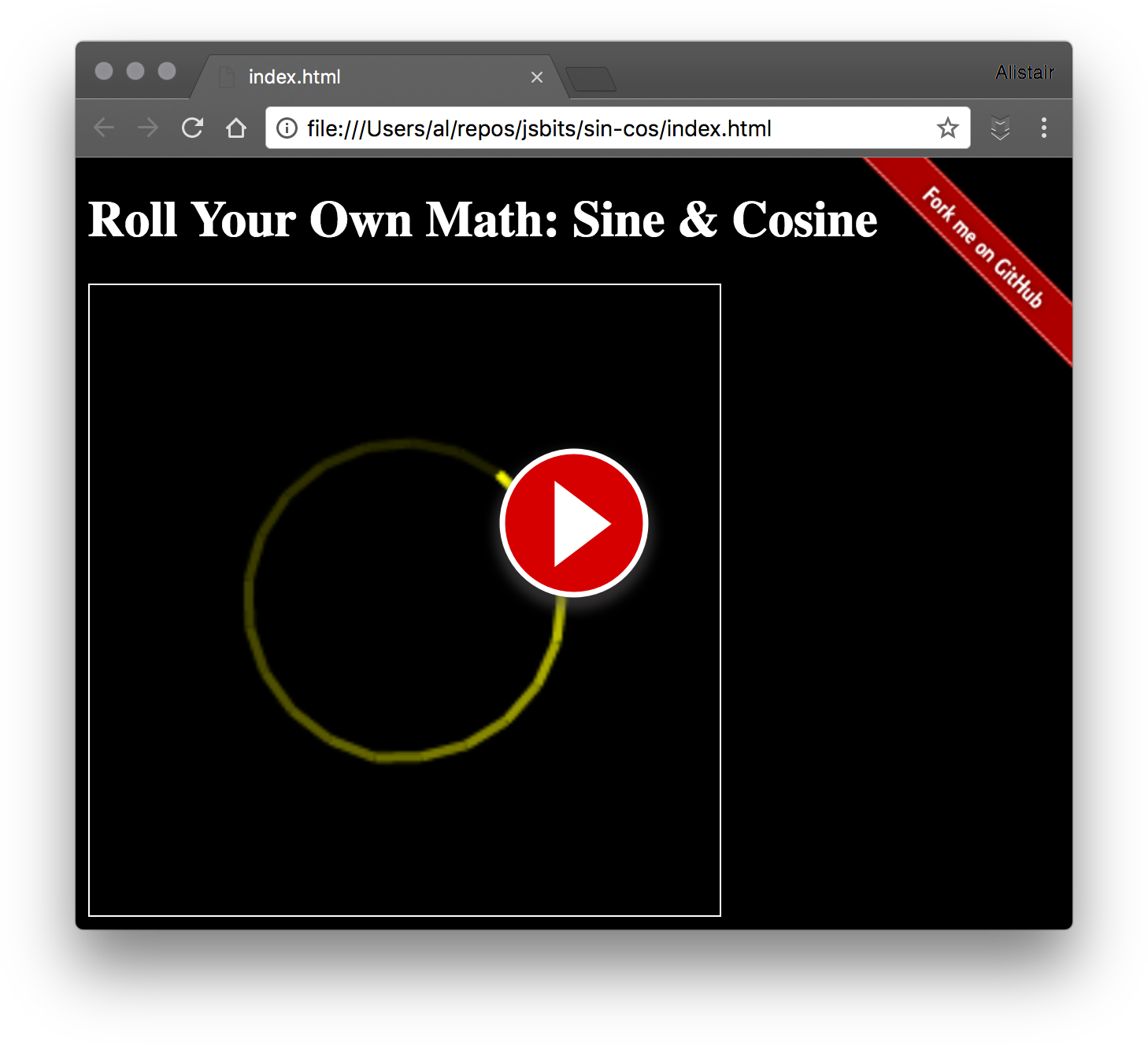## Bad Weekend

I was having a bad weekend. I was frustrated with whatever I was working on during the week, and I had got it into my head that I was possibly the worst programmer I had ever met.

(I don’t know about you, but find it pretty easy to beat myself up if I can’t figure out “the next best thing” without trying.)

So the second stupid thing I got into my head, was that the only solution to overcoming my woes was to understand how Sine and Cosine worked, as quickly as possible.

## The Rules

I felt like if I could re-create the Sine and Cosine functions using only simple math operators, then I would “know” where the magic was, thank God.

• Add `+`
• Subtract `-`
• Multiply `*`
• Divide `/`

## The Research

I hit up all the usual suspects: Wikipedia, MathOverflow, and Reddit Mathematics; and as usual, I got assaulted with more indecipherable squirrely symbols.

Surprisingly, Quora seemed to have a good start on things. Math Samurai “David Joyce” went to the effort of explaining the Math quite clearly.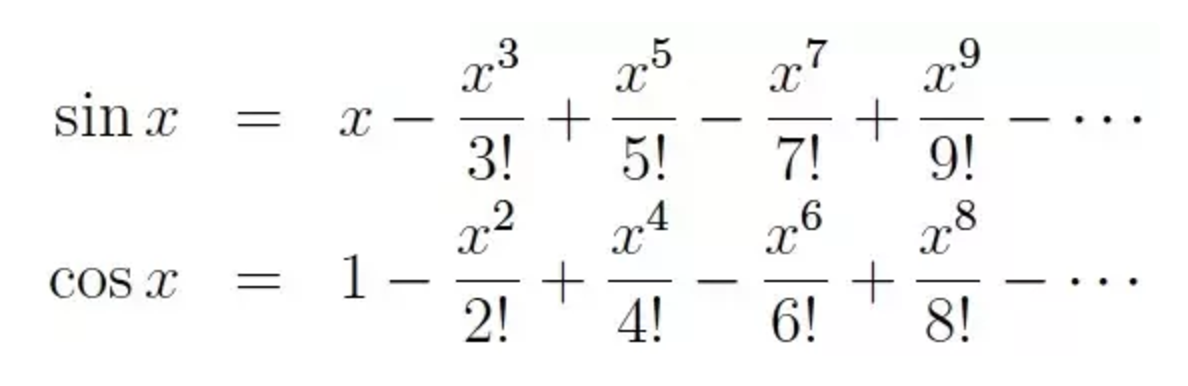But I still didn’t get it.

Eventually, I stumbled upon a treasure-trove of intuitive math information from Kalid Azad at BetterExplained.com. (You have to read this guy’s website, it’s AMAZING!). His article on the Intuitive Understand of Sine Waves was the exact information I needed. I had to re-read it, a lot, but it finally clicked!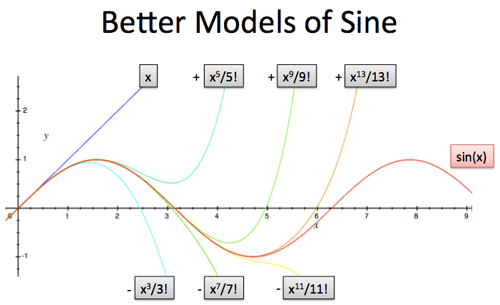## Learning a Few Things

It became apparent that rolling-my-own Sine and Cosine functions with basic math operators would require “rolling-a-few-more-of-my-own” math functions than I had expected.

Taking account of the damage:

• Powers
• Factorials
• Infinite Series
• Restoring Forces

To truly understand how Sine and Cosine work, it will help to first understand what these math functions do. Let us take a quik look.

## Understanding Powers

Probably the easiest to understand is the power. A Power is a number, multiplied by itself. The number that we want to multiply is called the “base”. And the value that we want to multiply it by, is called the “exponent” or the “power”.

Powers are often represented in code with a circumflex character, for example, `base ^ power` or `2 ^ 3`, which is the same as saying “two-to-the-third” or “2 to the 3rd power”.

Lets run through some examples: `2^1` (two-to-the-power-of-one), is “two”; which is the same as saying: two times one equals two.

``````2 * 1 = 2
``````

And `2^2` (two-to-the-power-of-two), is the same as saying: two times two.

``````2 * 2 = 4
``````

And `2^3` (two-to-the-power-of-three), is the same as saying: two times two, times two.

``````2 * 2 * 2 = 8
``````

You may know that the values of binary digits, (the 1’s and 0’s that make up the base language of computers), are all powers of two:

``````2^1 = 2
2^2 = 4
2^3 = 8
2^4 = 16
2^5 = 32
2^6 = 64
2^7 = 128
2^8 = 256
``````

Putting this together in JavaScript might look something like this:

``````// Example: let base = 2 and exponent = 3
const power = (base, exponent) => {

// Initialize return value with 2
// Eg: let val = 2
let val = base

// Initialize counter
let i = 0

// While counter is lower than exponent
// Eg: while (0 < 2)...
while (i < exponent - 1) {

// Multiple the return value by the base
// Eg: 2 * 2 = 4 (val is now 4)
val *= base
i += 1

// Repeat until we have multiplied the base
// by "n"-times (where "n" is the exponent)
}

return val
}
``````

The next time we iterate the while loop, multiplying the base by the exponent, our values will have changed like so:

``````// While counter is lower than exponent
// Eg: while (1 < 2)...
while (i < exponent - 1) {

// Multiple the return value by the base
// Eg: 2 * 2 * 2 = 8 (val is now 8)
val *= base
i += 1
}

// Exit the loop because `i = exponent - 1`)
// Eg: 2 = 3 - 1
``````

Sometimes it is hard to visualize what the loop is doing. One way to make things clearer is to read out the values of the loop; that code might look something like this:

``````const power = (base, exponent) => {
let val = base
let i = 0

// LOG OUTPUT
console.log(i, val)

while (i < exponent - 1) {
val *= base
i += 1

// LOG OUTPUT
console.log(i, val)
}
}

power(2, 8)
``````

The code above would log something like the following output:

``````i, val
0, 2
1, 4
2, 8
3, 16
4, 32
5, 64
6, 128
7, 256
``````

## Understanding Factorials

With “Power” out of the way, let us take a peek at Factorial. A “Factorial” is usually represented with a exclamation, for example, `5!`, which is the same as saying “five-factorial”.

The factorial is an integer number (a whole number), multiplied by all of the integer numbers lower than it. For example, the calculation for `3!` (three-factorial) would look like this:

``````3! = 3 * 2 * 1 = 6
``````

In turn, the calculation for `5!` (five-factorial) would look like this:

``````5! = 5 * 4 * 3 * 2 * 1 = 120
``````

Here is one way to calculate the factorial in JavaScript. I have added console logs to the code, so we can get a better idea of what is going on:

``````const factoral = n => {
let val = n

console.log(n, val)

while (n > 2) {
val *= n - 1
n -= 1

console.log(n, val)
}

return val
}

const f5 = factoral(5)

console.log(`5! = \${f5}`)
``````

The output looks something like this:

``````i, val
5, 5
4, 20
3, 60
2, 120
5! = 120
``````

Breaking this down, we can see that this function iterates backwards. As with the Power function, we initialize our value outside of the loop, so the `while` statement does not count down to 1.

``````const factoral = n => {
let val = n
// Eg: val = 5

// The factorial for 1 is "1", so we
// gain nothing from solving for "1"
while (n > 2) {
val *= n - 1
/* Eg:
n-1 = 4
val = val * 4
val = 5 * 4
val = 20
*/

// Count backwards from 5 to 2.
n -= 1
}

return val
}

const f5 = factoral(5)
``````

## Infinite Series

Understanding the Infinite Series was where things got exciting for me. The concept is pretty simple: a series is “the operation of adding infinitely many quantities, one after the other, to a given starting quantity.” - Wikipedia

In a simple sense, could express this like so:

``````sum = 1 + 2 + 3 + 4 + ... (to-infinity!)
``````

But we could also express a series of more complicated functions, for example, a series of Power functions:

``````sum = 1^2 + 2^2 + 3^2 + 4^2 + ... (to-infinity!)
``````

But the series does not have to use power functions; we could use anything we like.

### Why Infinite?

You might also be wondering what the “infinite” in “infinite series” means. Infinite in this sense is not to say we will try to calculate an infinite value! (That would be impossible). But the term “infinite-series” expresses the idea: that if we continued to determine the value for next step in our series, we would continue to find more values. We could keep running the function forever and get new results each time.

In this way, we might be able to think our Power-function is an infinite series and our Integer Factorial function as a finite series. The power keeps getting bigger each step we add, and the integer-factorial gets smaller until we run out of steps.

The infinite series that we will use to generate our Sine and Cosine waves will require us to stop and read out the value at some point. The point at which we will stop is called the “precision.”

Note: that if we let the series run, we will be forced to stop at a certain percision anyway, because we will run out of decimal places to divide into.

## Restoring Forces

A restoring force is a force that pushes back on an impulse, eventually regaining equilibrium. For example: if you are driving a car west, against a steadily increasing easterly wind, you will have to put your foot down harder and harder on the pedal to maintain your speed. In this way, the wind is a restoring force.

Perhaps a better example is gravity. The equilibrium of a ball sitting on the ground gets broken by the “impulse” of throwing a ball into the air, and then “restored” by the force of gravity pulling it back to the earth.

We need a restoring force to generate a Sine wave.

## Generating a Sine Wave

Khalid’s post on the Inuitive Understanding of Sine Waves is particularly helpful in understanding how to put the calculations together to generate a Sine Wave. We could express the restoring-force calculation in pseudo code like this:

``````y = x - (x^3 / 3!) + (x^5 / 5!) - (x^7 / 7!) ... (to infinity!)
``````

In the pseudo code example, you can see that we calculate a function in an infinite series, but pay attention to the plus `+` and minus `-`. The first time we run the function we subtract the result, and the second time we run the function we add the resulting value, and we keep going like this, back and forth, over and over; creating our restorative force.

We know that each time we raise a number to the power, it gets larger, and so our function returns an increasingly greater number as the series continues. And we also know that the factorial produces a larger number, as its initial value gets raised too. Does this mean the number gets larger?

No: because the series flips between addition and subtraction of the result, so the number is continually refined (until we reach precision). As we increase the precision (the number of steps in the series), we change how the restorative force effects the value.

If we run the psuedo code above in the NodeJS console, we can watch the impulse and the restorative force in action as we add each step in the series.

``````let x = 2
let y = x - (x^3 / 3!) + (x^5 / 5!) - (x^7 / 7!)

> 2 - (pow(2, 3) / fact(3))
0.6666666666666667
> 2 - (pow(2, 3) / fact(3)) + (pow(2, 5) / fact(5))
0.9333333333333333
> 2 - (pow(2, 3) / fact(3)) + (pow(2, 5) / fact(5)) - (pow(2, 7) / fact(7))
0.9079365079365079
``````

We can look at the values of the calculations we did above in this following table. You can see that as the series increases, and the powers and factorials increase, the sum value decreases! So as we raise the precision, the impulses and restorative forces decrease; refining the value by smaller and smaller amounts.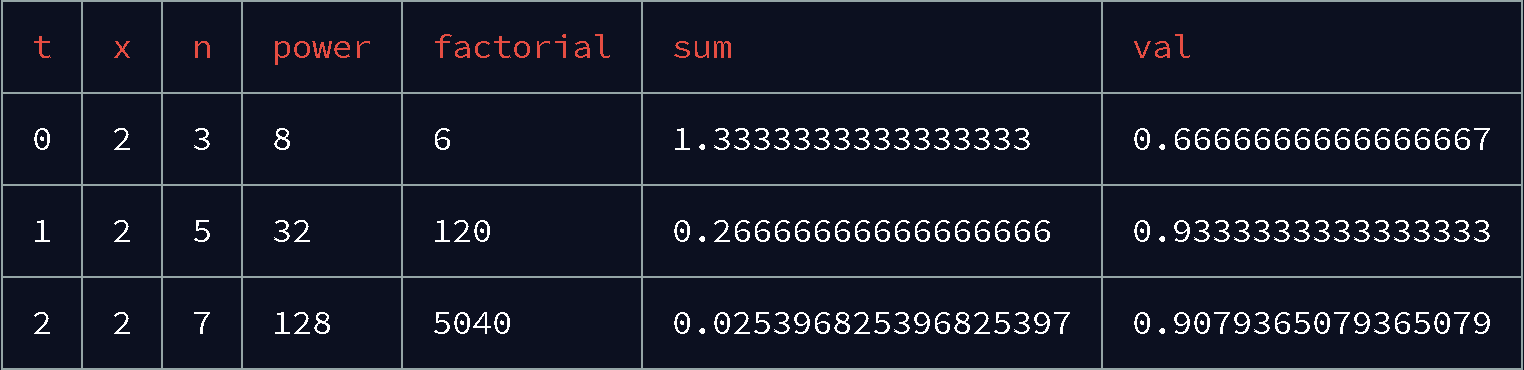So this back-and-forth is what generates the sine wave. And the more back-and-forth we have, (the more steps of precision we have), the longer our Sine wave extends.

This following CodePen plots the precision from 0 to 10. As the precision increases, and the steps in our series increase, we have more and more restorative forces acting upon the value, and so the wave “gets wavier” the more preceision we add.

See the Pen Roll Your Own Math - Sine by f1lt3r (@F1LT3R) on CodePen.

The important thing to consider here is that the longer the sine wave is that we want to plot, the higher the precision (steps in our series) will need to be in order for the wave to be pulled back across the center line. At some point, our wave will eventually escape gravity and shoot off the chart.

This concept might be a little clearer if we plot the precision of Sine and Cosine together as if we are trying to draw a circle. This following image plots precision 1 - 10. As the precision increases, the line progresses further around the circle before escaping. This plot also makes it easier to see the effect of ending the series calculation on a plus `+` versus a minus `-`, as you can see the line shoot off towards the top or bottom of the image respectively.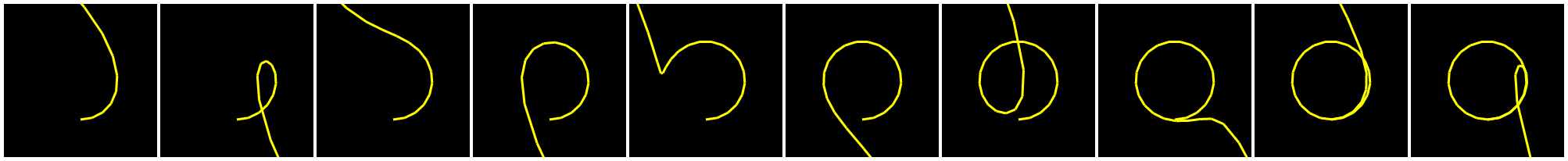We can see that it takes about 8 steps in our series to complete the circle. With need for increased precision in mind we might ask the question:

“How many steps in my series do I need to calculate a Sine wave of n-length?”

Fortunately we do not have to answer this question. We can use a little trick. We know that the 360 degrees of a circle are made up of approximately `~6.283` radians. So the value of `x` at `2π`, is the same as the value of `x` at `0`. So rather than increasing the precision of our series, we can confine the value of `x` to `2π`.

``````const series = (x, offset, precision) => {
// Constrain x to PI * 2 so that we don't need
// to add more precision as x increases
x %= PI2
}
``````

## Making Circles

Putting things together we can create a function that executes our series to a given precision.

``````const series = (x, offset, precision) => {
// Constrain x to PI * 2 so that we don't need
// to add more precision as x increases
x %= PI2

// Count the steps with `n`
let n = 0

// Set the impulse force
let val = x

// Until we reach our precision...
while (n < precision) {
// Calculate the step: [3, 5, 7, ...], [2, 4, 8, ...]
const step = offset + (n * 2)

// Calculate the sum for the current step in the series
const pow = power(x, step)
const fact = factorial(step)
const sum = pow / fact

// Apply the sum to the value as either an
// Impulse Force or Restorative Force
if (n % 2) {
val += sum
} else {
val -= sum
}

n += 1
}

// Return the value after reaching our desired precision
return val
}
``````

This `series()` function gives us everything we need to create our `sin()` function. Oue sine function just calls `series()` with the relevant values. To generate a sine wave, we set the offset to 3 and pass the value of x.

``````const sin = x => {
const sineOffset = 3
const value = series(x, sineOffset, precision)
return value
}
``````

### What about Cosine?

Cosine is slightly different. To calculate Cosine we need to make 2 changes to our series. Firstly, the step value is phase-shifted. To provide the shift in phase, our step values for Sine are `[3, 5, 7, ...]` and the step values for Cosine are `[2, 4, 6, ...]` and so-on.

Secondly, we do need an impulse force. Our Sine wave starts with an `x` of `0` but our Cosine is phase-shifted to 1. This means we need to add the following code to our `series()` function.

``````const series = (x, offset, precision) => {
// When calculating Cosine, contrain the value
// to `1` (no initial impulse)
if (offset === 2) {
val = 1
}
}
``````

Now we can interact with our `series()` function to create our Cosine function.

``````const cos = x => {
const cosineOffset = 2
return series(x, cosineOffset, precision)
}
``````

Now we have everything we need to make circles!

1. A Power function
2. A Factorial function
3. A Series method that can execute to a given precision
4. Sine and Cosine functions that can run a series

So let’s plot a circle with our custom `sin()` and `cos()` functions.

``````const mag = 50
const rate = 0.3
let t = 0

const draw = () => {
t += rate

const x = centerX + (sin(t) * mag)
const y = centerY + (cos(t) * mag)

plot(x, y)
}

setInterval(draw, 40)
``````

Here is a CodePen example of all these functions together in action:

See the Pen Roll Your Own Math - Sine & Cosine (Circle) by f1lt3r (@F1LT3R) on CodePen.

So that is how to Roll Your Own Sine and Cosine.

I must say a big “Thank you!” to Khalid from BetterExplained.com who’s blog post helped me understand how the math works.

Thoughts, comments, ideas? Let me know what you think.

```       ___ _ _  ____________
___  | __/ | ||_   ___   _ \
/___/ | _|| | |__| | |_ \   /
|_| |_|____|_||___/_|_\```